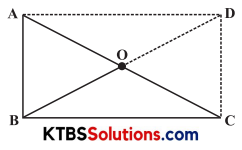# KSEEB Solutions for Class 8 Maths Chapter 3 Understanding Quadrilaterals Ex 3.4

You can Download KSEEB Solutions for Class 8 Maths Chapter 3 Understanding Quadrilaterals Ex 3.4 Questions and Answers helps you to revise the complete syllabus.

## KSEEB Solutions for Class 8 Maths Chapter 3 Understanding Quadrilaterals Ex 3.4

Question 1.
State whether True or False:

1. All rectangles are squares.
2. All rhombuses are parallelograms.
3. All squares are rhombuses and also rectangles.
4. All squares are not parallelograms.
5. All kites are rhombuses.
6. All rhombuses are kites.
7. All parallelograms are trapeziums.
8. All squares are trapeziums.

Solution:

1. False
2. True
3. True
4. False
5. False
6. True
7. True
8. TrueQuestion 2.
Identify all the quadrilaterals that have.

1. four sides of equal length.
2. four right angles.

Solution:

1. The quadrilateral is either a Rhombus or a Square.
2. The quadrilateral is either a Square or a Rectangle.

Question 3.
Explain how a square is.

2. a parallelogram
3. a rhombus
4. a rectangle

Solution:

1. A square is a four-sided closed figure, so it is a quadrilateral.
2. In a square, both pairs of opposite sides are equal. Hence, it is a parallelogram.
3. A square is a rhombus because all sides of a square are equal.
4. A square is a rectangle because its opposite sides are equal and all angles are 90° each.Question 4.

1. bisect each other.
2. are perpendicular bisectors of each other.
3. are equal.

Solution:

1. The diagonals of a parallelogram, rectangle, rhombus and square bisect each other.
2. The diagonals are perpendicular bisectors in squares and rhombus.
3. The diagonals are equal in square and rectangle.

Question 5.
Explain why a rectangle is a convex quadrilateral.
Solution:
Both of its diagonals lie in its interior.Question 6.
ABC is a right-angled triangle and O is the midpoint of the side opposite to the right angle. Explain why O is equidistant from A, B, and C. (The dotted lines are drawn additionally to help you).Solution:
OB is produced to D such that OB = OD